# VLOOKUP In VBA – With Examples

In my earlier post, I had written about VLookUp in Excel. It was a massive post of around 2500 words, it explains most of the things about vertical look up function in excel. Today’s post is an extension to that post and here we will understand how to apply a VLookUp in VBA.

If you haven’t read that post then I would strongly recommend you to read that post before going any further. [Read Here]

Assuming that you have basic knowledge of VLOOKUP function we will move further.

Note: To perform these programs yourself, you may need to enable macros in excel. Read this post to know how to do this.

### Syntax of VBA VLOOKUP:

You can use VLookUp in macros by following any of the below ways:

1. `Application.VLOOKUP(lookup_value, table_array, column_index, range_lookup)`

Or

2. `Application.WorksheetFunction.VLOOKUP(lookup_value, table_array, column_index, range_lookup)`

Note: If you are searching for something similar to VLookUp function for Access then probably you should use DLOOKUP.

### 5 Examples of Using VLOOKUP in VBA:

Now let’s move to some practical examples of using VLookUp in VBA codes.

Example 1:Using VLookUp find the monthly salary of “Justin Jones” from the below table. Display the salary using a dialog box.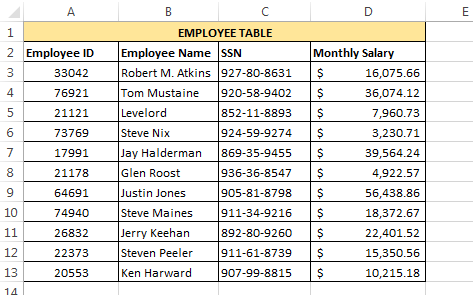Below is the code for this:

`Sub FINDSAL()Dim E_name As StringE_name = "Justin Jones"Sal = Application.WorksheetFunction.VLookup(E_name, Sheet1.Range("B3:D13"), 3, False)MsgBox "Salary is : \$ " & SalEnd Sub`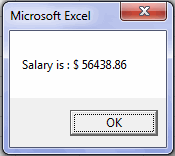Explanation: In this code we have used a variable ‘`E_name`’ to store the employee name whose salary is to be fetched. After this we have simply supplied the employee name and other required arguments to the VLOOKUP and it returns the salary of the corresponding Employee.

Example 2: Now make the above program a little customisable by accepting the Employee name from user. If user enters any Employee name that is not present in the table then the program should be able to convey this clearly to the user.

To accomplish this we can use the below code:

`Sub FINDSAL()On Error GoTo MyErrorHandler:Dim E_name As StringE_name = InputBox("Enter the Employee Name :")If Len(E_name) > 0 Then Sal = Application.WorksheetFunction.VLookup(E_name, Sheet1.Range("B3:D13"), 3, False) MsgBox "Salary is : \$ " & SalElse MsgBox ("You entered an invalid value")End IfExit SubMyErrorHandler:If Err.Number = 1004 Then MsgBox "Employee Not Present in the table."End IfEnd Sub`

Explanation: In this code we are accepting the user input using an InputBox function. If the Employee name entered by the user is found, then VLookUp returns its corresponding salary. However if the employee name is not present in the table then VLOOKUP throws a “1004 Error”.

And, we have created an error handler to catch such cases for conveying the user that entered employee name doesn’t exist.

Example 3:In this example we will try to write a code that adds the Department field from the Employee Table 1 to our old Employee Table.As you can see that in both these tables there is only one common column i.e. `Employee_ID`. So, in this case we will have to apply the VLookUp based on the Employee ID.

Below is the code to do this:

`Sub ADDCLM()On Error Resume NextDim Dept_Row As LongDim Dept_Clm As LongTable1 = Sheet1.Range("A3:A13") ' Employee_ID Column from Employee tableTable2 = Sheet1.Range("H3:I13") ' Range of Employee Table 1Dept_Row = Sheet1.Range("E3").Row ' Change E3 with the cell from where you need to start populating the DepartmentDept_Clm = Sheet1.Range("E3").ColumnFor Each cl In Table1 Sheet1.Cells(Dept_Row, Dept_Clm) = Application.WorksheetFunction.VLookup(cl, Table2, 2, False) Dept_Row = Dept_Row + 1Next clMsgBox "Done"End Sub`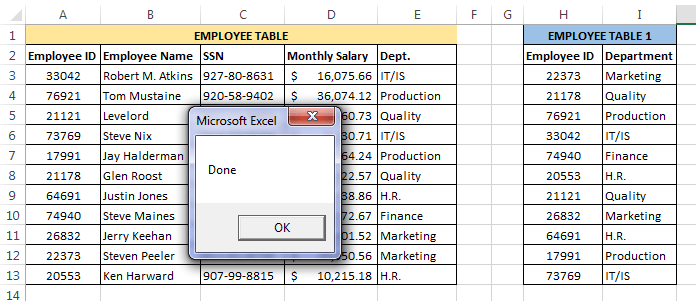Explanation: This code takes each ‘`lookup_value`’ from the Employee ID field (one at a time), looks up its corresponding Department and then populates the corresponding department value at appropriate place.

Please note that in this code we have just pasted the result of VLookUp formula, and not the VLookUp formula itself (Refer Example 5).

Example 4:In this example we will try to write a code that displays all the details of an Employee from the Employee table (as shown below) when its Employee ID is entered.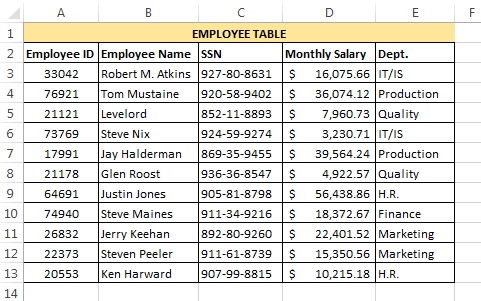Below is the code that can accomplish this:

`Sub FETCH_EMP_DETAILS()On Error GoTo MyErrorHandler:Dim E_id As LongE_id = InputBox("Enter the Employee ID :")Det = "Employee ID : " & Application.WorksheetFunction.VLookup(E_id, Sheet1.Range("A3:E13"), 1, False)Det = Det & vbNewLine & "Employee Name : " & Application.WorksheetFunction.VLookup(E_id, Sheet1.Range("A3:E13"), 2, False)Det = Det & vbNewLine & "Employee SSN : " & Application.WorksheetFunction.VLookup(E_id, Sheet1.Range("A3:E13"), 3, False)Det = Det & vbNewLine & "Monthly Salary : " & Application.WorksheetFunction.VLookup(E_id, Sheet1.Range("A3:E13"), 4, False)Det = Det & vbNewLine & "Department : " & Application.WorksheetFunction.VLookup(E_id, Sheet1.Range("A3:E13"), 5, False)MsgBox "Employee Details : " & vbNewLine & DetExit SubMyErrorHandler:If Err.Number = 1004 Then MsgBox "Employee Not Present in the table."ElseIf Err.Number = 13 Then MsgBox "You have entered an invalid value."End IfEnd Sub`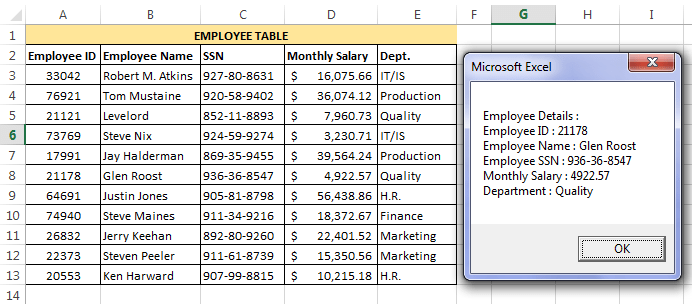Explanation:In this example we have asked user to enter the `Employee Id` and then we have used multiple VLookUp Statements and concatenated their outputs to show all the details in a single message box.

Example 5:Redo the example 3 but this time paste the whole VLookUp formula instead of pasting only the result.Below is the code for doing this:

`Sub ADDCLM()On Error Resume NextDim Dept_Row As LongDim Dept_Clm As Longctr = 0Table1 = Sheet1.Range("A3:A13") ' Employee_ID Column from Employee tableTable2 = Sheet1.Range("H3:I13") ' Range of Employee Table 1Dept_Row = Sheet1.Range("E3").Row ' Change E3 with the cell from where you need to start populating the DepartmentDept_Clm = Sheet1.Range("E3").ColumnFor Each cl In Table1 Sheet1.Cells(Dept_Row, Dept_Clm).FormulaR1C1 = "=VLOOKUP(RC[-4], R3C8:R13C9, 2, False)" Dept_Row = Dept_Row + 1 ctr = ctr + 1Next clMsgBox "Done"End Sub`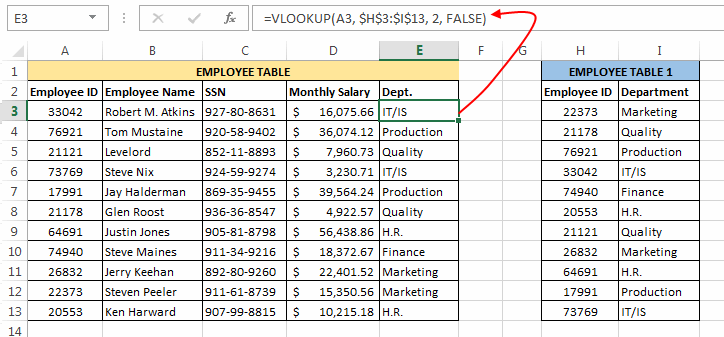Explanation:This code is very similar with the one that we have discussed in Example 3, the only difference between these formulas is that here we are copying the VLookUp formula directly in the cells.

In this code we have applied the VLOOKUP in R1C1 form. So, the formula =VLOOKUP(RC[-4], R3C8:R13C9, 2, False) means =VLOOKUP(<4 cells to the left of current cell>, <Range of Employee Table 1>, <column to be fetched>, <exact match>).

One thing that is worth to note here is : the square brackets ( [ ] ) in your R1C1 formula indicate that you are specifying a relative range. If you want to specify an absolute range, you need to specify the R1C1 cells without brackets; e.g. R3C8:R13C9.

So, this was all about VBA VLookUp.About Ankit Kaul

Ankit is the founder of Excel Trick. He is tech Geek who loves to sit in front of his square headed girlfriend (his PC) all day long. :D. Ankit has a strong passion for learning Microsoft Excel. His only aim is to turn you guys into 'Excel Geeks'.

1.Rahim Zulfiqar Alisays

Simply Awesome 🙂 Thanks

•Ankit Kaulsays

It’s my Pleasure! Thanks for reading :).

2.Rahim Zulfiqar Alisays

I have created a webpage https://sites.google.com/site/vlookupweek2012/
Its related to VLOOKUP with more examples. You can share on your Blog. You can also re-write if you want. Thank you

•Ankit Kaulsays

Thanks for sharing the URL. I will definitely have a look at it.

•Rudra Sharmasays

Hi Rahim,
3.amitsays
•Ankit Kaulsays